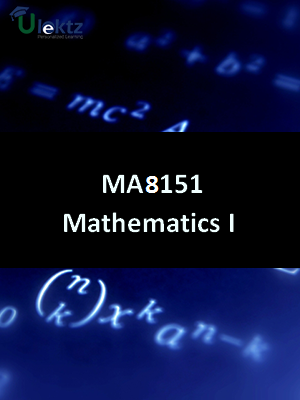uLektz apps

•My WalletMy Order
•My Profile
•My Connections
•My Books
•My Videos
•My Tests
•My Calender
•My Messages
•My Shopping Cart
•My Orders
•Account Settings
•Help

uLektz apps

# Book Details# Engineering Mathematics - I

Course Code:MA8151

Author:uLektz

University:

Regulation:2017

Categories:General Engineering

Format :ePUB3 (DRM Protected)

Type :eBook

FREE

Description :Engineering Mathematics - I of MA8151 covers the latest syllabus prescribed by Anna University, Tamil Nadu for regulation 2017. Author: uLektz, Published by uLektz Learning Solutions Private Limited.

Note : No printed book. Only ebook. Access eBook using uLektz apps for Android, iOS and Windows Desktop PC.

##### Topics
###### UNIT I DIFFERENTIAL CALCULUS

1.1 Representation of functions - Limit of a function - Continuity

1.2 Derivatives

1.3 Differentiation rules - Maxima and Minima of functions of one variable

###### UNIT II FUNCTIONS OF SEVERAL VARIABLES

2.1 Partial differentiation

2.2 Homogeneous functions and Euler’s theorem

2.3 Total derivative - Change of variables

2.4 Jacobians - Partial differentiation of implicit functions

2.5 Taylor’s series for functions of two variables

2.6 Maxima and minima of functions of two variables - Lagrange’s method of undetermined multipliers

###### UNIT III INTEGRAL CALCULUS

3.1 Definite and Indefinite integrals - Substitution rule

3.2 Techniques of Integration - Integration by parts - Trigonometric integrals - Trigonometric substitutions - Integration of rational functions by partial fraction - Integration of irrational functions - Improper integrals

###### UNIT IV MULTIPLE INTEGRALS

4.1 Double integrals

4.2 Change of order of integration-Double integrals in polar coordinates

4.3 Area enclosed by plane curves

4.4 Triple integrals - Volume of solids

4.5 Change of variables in double and triple integrals

###### UNIT V DIFFERENTIAL EQUATIONS

5.1 Higher order linear differential equations with constant coefficients - Method of variation of parameters

5.2 Homogeneous equation of Euler’s and Legendre’s type - system of simultaneous linear differential equations with constant coefficients - Method of undetermined coefficients

### Related Books